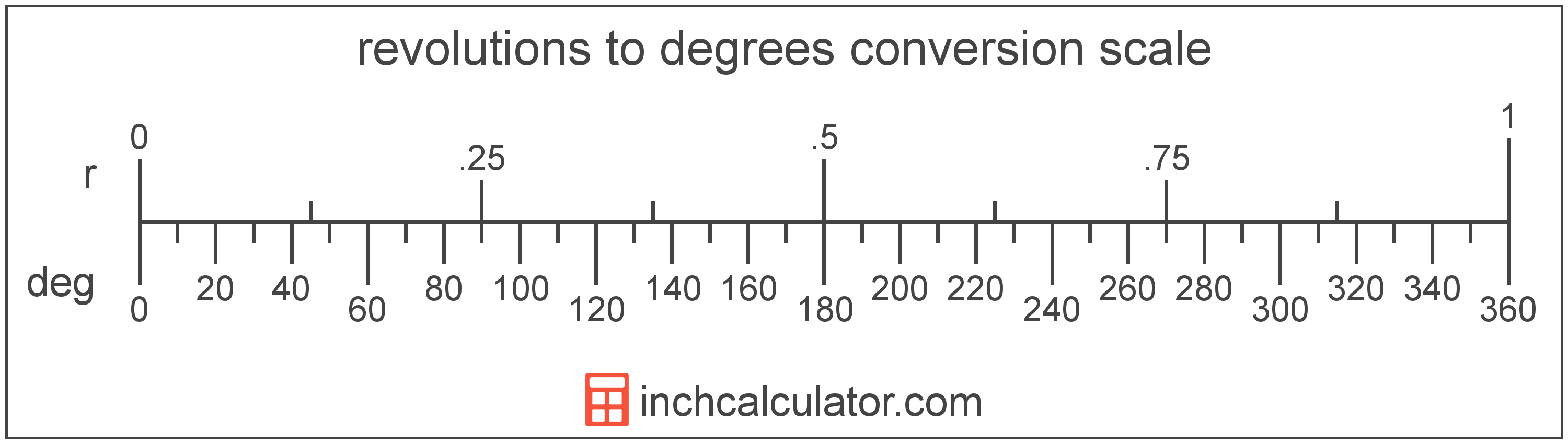# Degrees to Revolutions Converter

Enter the angle in degrees below to get the value converted to revolutions.

Results in Revolutions:1° = 0.002778 r

Do you want to convert revolutions to degrees?

## How to Convert Degrees to Revolutions

To convert a measurement in degrees to a measurement in revolutions, divide the angle by the following conversion ratio: 360 degrees/revolution.

Since one revolution is equal to 360 degrees, you can use this simple formula to convert:

revolutions = degrees ÷ 360

The angle in revolutions is equal to the angle in degrees divided by 360.

For example, here's how to convert 500 degrees to revolutions using the formula above.
revolutions = (500° ÷ 360) = 1.388889 r## What is a Degree?

A degree is a measure of angle equal to 1/360th of a revolution, or circle. The number 360 has 24 divisors, making it a fairly easy number to work with. There are also 360 days in the Persian calendar year, and many theorize that early astronomers used 1 degree per day.

The degree is an SI accepted unit for angle for use with the metric system. A degree is sometimes also referred to as a degree of arc, arc degree, or arcdegree. Degrees can be abbreviated as °, and are also sometimes abbreviated as deg. For example, 1 degree can be written as 1° or 1 deg.

Degrees can also be expressed using arcminutes and arcseconds as an alternative to using the decimal form. Arcminutes and arcseconds are expressed using the prime (′) and double-prime (″) characters, respectively, although a single-quote and double-quote are often used for convenience.

One arcminute is equal to 1/60th of a degree, and one arcsecond is equal to 1/60th of an arcminute.

Protractors are commonly used to measure angles in degrees. They are semi-circle or full-circle devices with degree markings allowing a user to measure an angle in degrees. Learn more about how to use a protractor or download a printable protractor.

## What is a Revolution?

A revolution, or turn, is equal to 1 rotation around a circle, or 360°. Revolutions are commonly used to measure the speed of rotation, for example when measuring the revolutions per minute (RPM) of a vehicle's engine.

A revolution is sometimes also referred to as a turn, cycle, or complete rotation. Revolutions can be abbreviated as r, and are also sometimes abbreviated as rev or cyc. For example, 1 revolution can be written as 1 r, 1 rev, or 1 cyc.

## Degree to Revolution Conversion Table

Table showing various degree measurements converted to revolutions.
Degrees Revolutions
0.002778 r
0.005556 r
0.008333 r
0.011111 r
0.013889 r
0.016667 r
0.019444 r
0.022222 r
0.025 r
10° 0.027778 r
20° 0.055556 r
30° 0.083333 r
40° 0.111111 r
50° 0.138889 r
60° 0.166667 r
70° 0.194444 r
80° 0.222222 r
90° 0.25 r
100° 0.277778 r
200° 0.555556 r
300° 0.833333 r
400° 1.1111 r
500° 1.3889 r
600° 1.6667 r
700° 1.9444 r
800° 2.2222 r
900° 2.5 r
1,000° 2.7778 r

## References

1. Collins Dictionary, Definition of 'degree', https://www.collinsdictionary.com/us/dictionary/english/degree